# Evaluating Algebraic Expressions

Times up!
Times up! You may finish answering the current question and then proceed to your score!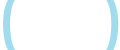Sorry, you have used all of your available hints for this lesson.
Practice Limit Reached

You've reached your daily practice limit of 12 questions.

Award InformationAwarded toQuestion HintChoose Family Member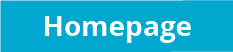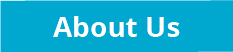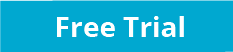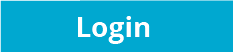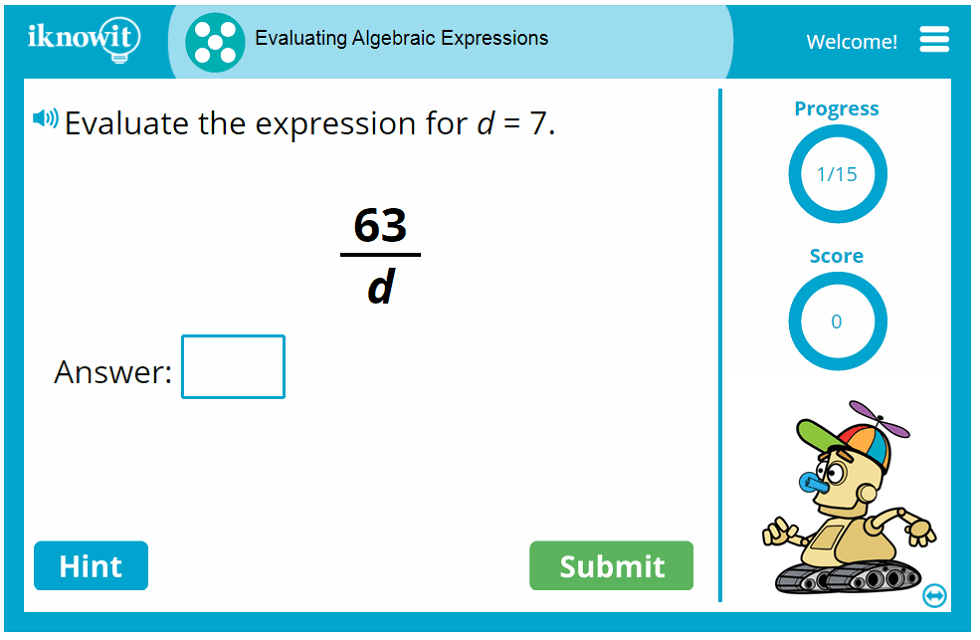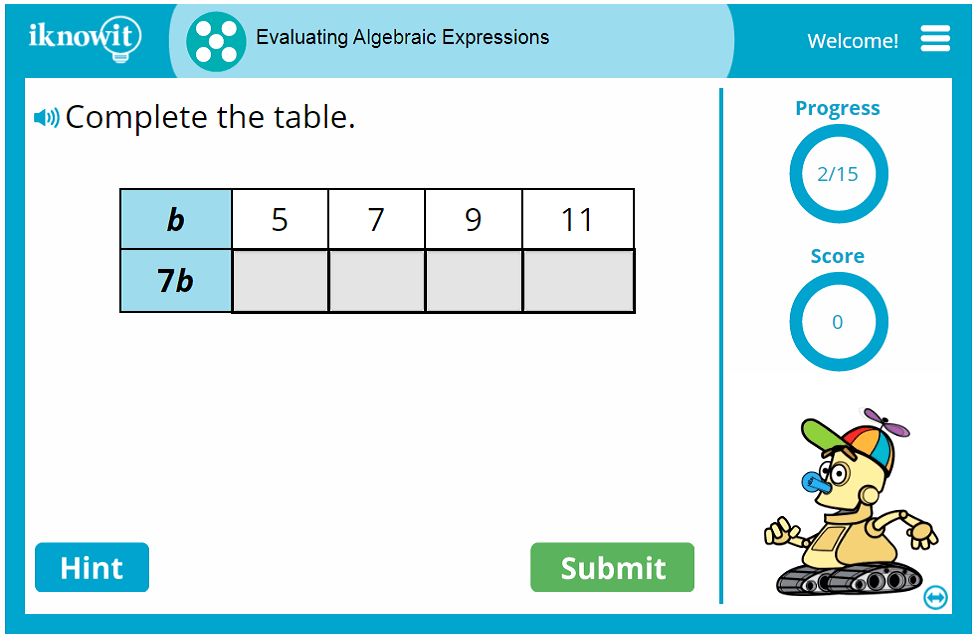## Algebraic Expressions Math Game

Practice evaluating basic algebraic expressions. This interactive math game will help students gain a greater understanding of basic algebra. In this online math lesson, students will practice the following skills:

* Evaluate the algebraic expression. (For example, evaluate the expression for: k = 6, 42/k)

* Complete the table to evaluate each algebraic expression. (For example, complete the expression when z = 2, z = 4, z = 6, and so on.)

* Evaluate the algebraic expression in word problem format. (For example, "If v = 7, what is 49/v?")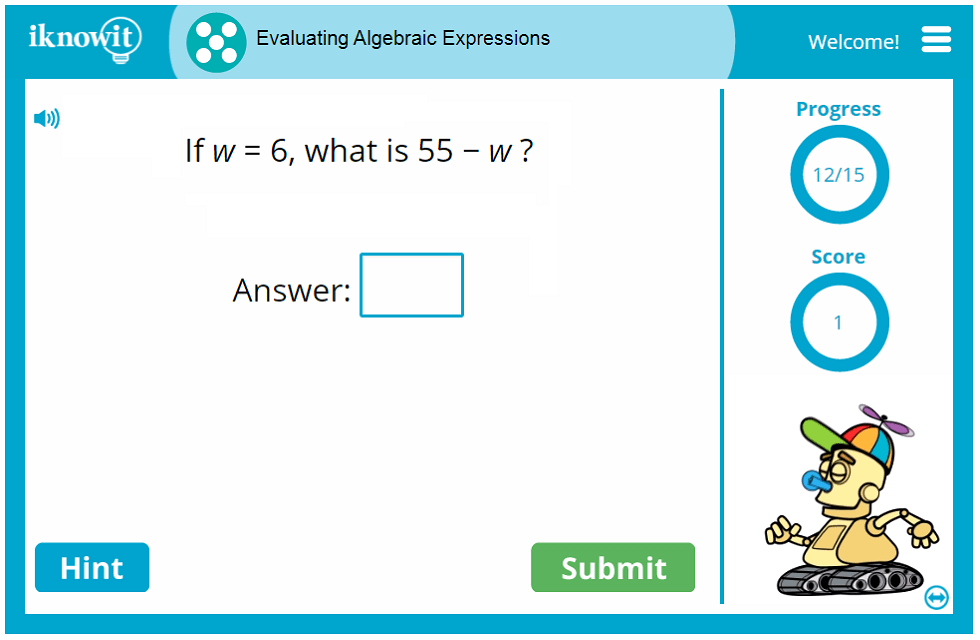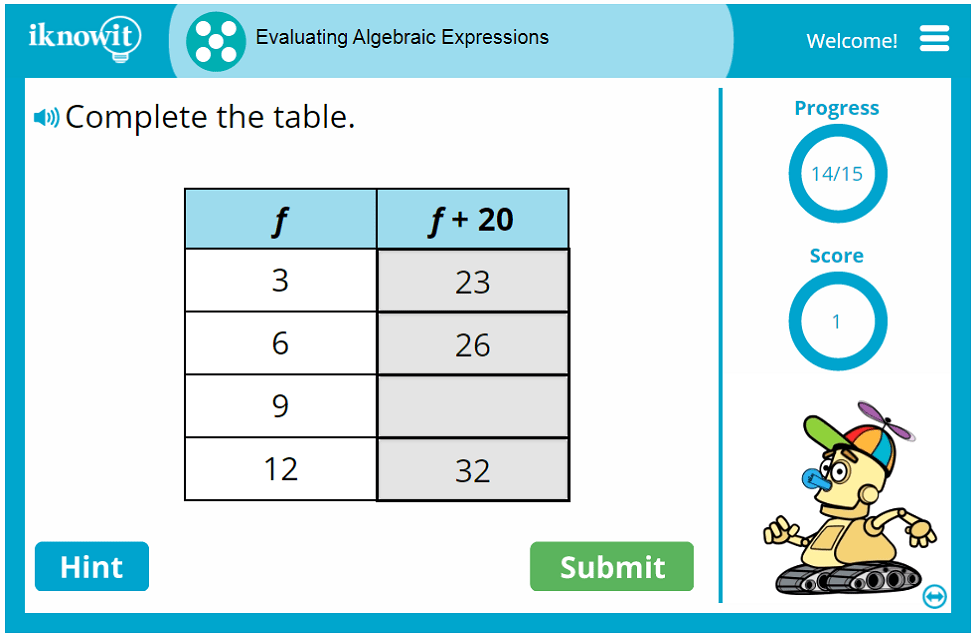## Practice Math, Play a Game

Students enjoy using iKnowit.com math lessons because it feels like they are playing a fun game. Learning becomes easy and entertaining. When a student gets a question correct, an animated character on the screen does a clever trick or even dances around! When the student answers a question incorrectly, he or she will be shown a detailed explanation, complete with an easy-to-understand graphic, that shows how to obtain the right answer next time.

If students need a little nudge in the right direction, they can opt for a hint, using the "Hint" icon. They will be given a clue to help them figure out the math problem. The teacher or parent has the ability to turn the hint mode on or off.

## Register for an Account to Access All Features

When you sign up for an iKnowit.com account, you will be able to use our helpful administrative features. You can set up a class roster and create an individual login for each of your students. Each time a student does math problems on iKnowit, you will be able to see the scores and which problems they missed.

When students log in to use the math program, they will have their own kid-friendly homepage. There is an "Assignments from the Teacher" tab on the top of the students' page, plus a recommendation of math topics to try out. Students won't see the grade levels of the math assignments so that you can choose the appropriate grade level for each of your students without them knowing if they are working at a different grade level.

As a teacher, you can modify which animations your students see. You can also decide whether to use the read-aloud audio option. In addition, you can decide how many hints a student should get in each math lesson.

We encourage you to explore the hundreds of math lessons we have available on iKnowit.com. Whether you're looking for place value games, addition and subtraction practice, or geometry lessons, we cover a wide variety of math topics. To see more math games for fifth grade, please visit our Fifth Grade Math page.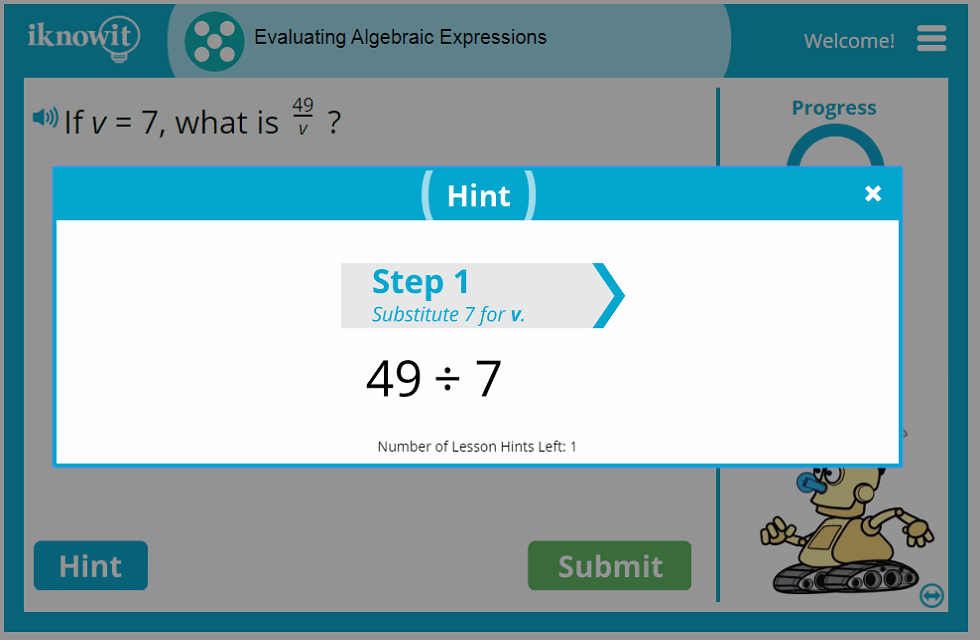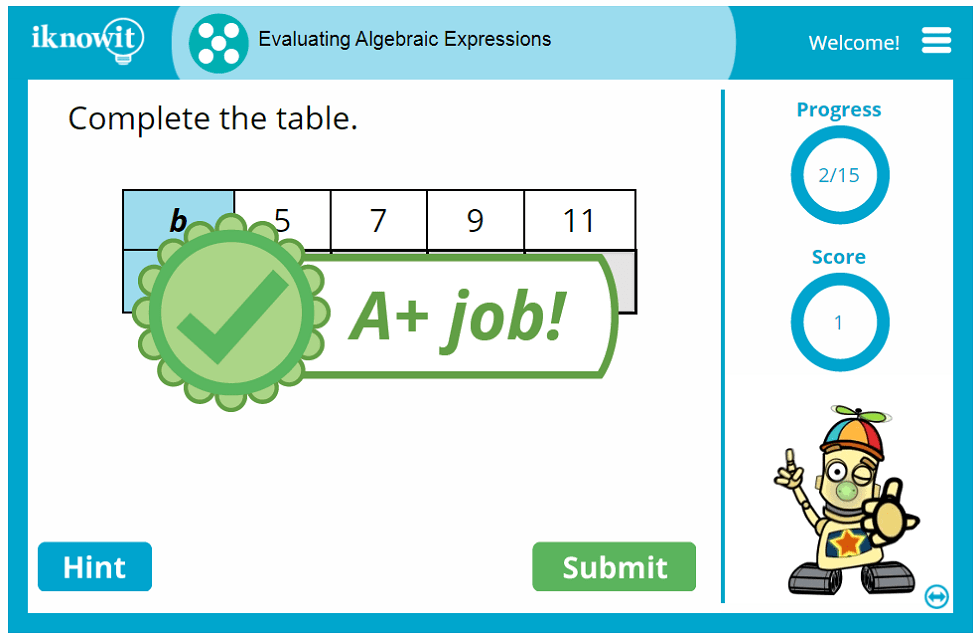## Level

This lesson is designated as Level E and is targeted toward fifth graders.

## Common Core Standard Alignment

5.OA.1
Algebraic Thinking and Operations: Interpret and Write Algebraic Expressions

Students must demonstrate an ability to use braces, parentheses, and brackets in numerical expressions. They should also be able to evaluate expressions that contain these symbols.

## You might also be interested in...

Length Conversions (Metric) (Level E)
Convert measurements using the metric system, including conversions between millimeters, centimeters, meters, and kilometers. The lesson includes word problems and drag-and-drop question formats.

Equivalent Fractions (Level E)
Make equivalent fractions by filling in the correct number for the numerator or denominator. Decide which fractions are equivalent to the given fraction. Pick which number sentence is true. Question formats include multiple choice.